Subscribe to Entrepreneur for \$5

# How is An Annuity Income Payout Calculated?

We recently wrote a piece showing how much income you can expect to receive every month from different types of annuities, including fixed, immediate income annuities and deferred income annuities....

By
This story originally appeared on Due

We recently wrote a piece showing how much income you can expect to receive every month from different types of annuities, including fixed, immediate income annuities and deferred income annuities. While that post is very useful to get a general idea of how much income you stand to receive based on how much you invest and for how long, those numbers are no more than estimations because there are many variables involved in the calculations and those variables are unlikely to agree perfectly with what your issuer will ultimately offer.

That's why it's important to learn how to calculate annuity income yourself to see if everything checks out in the contract or to figure out how much you actually need to save to receive the desired amount every month once you retire. In this post, we'll explore how to calculate annuity income in three different scenarios. You can also use these equations to calculate how much income you could generate for yourself (without purchasing an annuity) by investing your savings in stocks or other investment vehicles with a higher ROI but more risk.

Let's start by defining some key terms and variables we'll use to calculate annuity income.

## Key terms and variables

Before discussing the formulas used to calculate annuity payments, let's briefly define some key terms that we'll use throughout the following sections of the post.

### What is an annuity?

An annuity is a contract you sign with an insurance company in which you provide a premium in the form of a lump sum or periodic payments and, in exchange, the insurance company guarantees a series of periodic (usually monthly) payments once you retire either for a set number of years or for the rest of your life.

### Types of annuities

When calculating annuity income, the type of annuity determines which formula or equation to use. There are several types of annuities, including:

Each type of annuity has its own way of calculating income. In addition, you can personalize your annuity contract by adding special annuity riders that add more leverage, principal protection and guarantees. Most of these riders come with a fee that reduces the amount of income-generating capital you'll have at your disposal, and they also affect the rate the insurance company will offer.

### Annuitant

The person entering an annuity contract and who will receive the annuity payments.

### Annuitization or payout phase

Stage of an annuity contract when the insurance company makes the agreed payments to the annuitant.

### Accumulation phase

In the case of deferred annuities, this is the time between signing the contract and paying the agreed-upon premium (or premiums), and the beginning of the annuitization phase.

### Annuity rates (rm)

There are several different types of rates to consider with annuities. The rate the insurance company offers you is usually a nominal annual rate, not the actual annual rate you'll receive (which is called the effective rate). We'll refer to this rate as rm. In some cases, there may be a difference between the rate in the accumulation phase and the rate during the annuitization phase.

### Duration of the annuity (t)

The total number of years that the annuitization (or accumulation) lasts.

### Number of annual conversion or compounding periods (m)

This is the number of times per annum that you pay premiums in a fractioned annuity (one that pays more than once a year) during the accumulation phase or the number of times during the year that the insurance company makes payouts during the annuitization phase (usually 12).

### Total number of annuity conversions or compounding periods (p)

Most annuities pay out monthly, bimonthly or quarterly. The conversion periods are the total number of such payments you'll receive during the length of the annuitization phase or the number of premiums or contributions you're required to make during the entire accumulation phase. This is calculated simply as p = t * m.

### Monthly cash flow or annuity payments (Cm)

We'll use Cm to refer to the annuity payments we want to calculate and Cm,a for the periodic premiums we pay during the accumulation phase in some types of deferred annuities.

### Present Value of an Annuity (PVA or A0)

The current value of all future payments throughout the annuity. We can interpret this as the bulk sum you would have to pay the insurance company for them to guarantee a fixed income (Cm) at the proposed rate (rm) for a given number of years (t).

### Future Value of an Annuity after p conversion periods (FVA or Ap)

It's the added value of all annuity payments (or premiums) plus interests at a future date.

## How is annuity income calculated?

Calculating annuity income is actually very easy. Regardless of the type of annuity (immediate or deferred), all you need to know is the value of your investment the moment the annuitization begins, how long you'll receive steady income and the rate (be it fixed or variable) at which your investment grows over time.

In the case of immediate annuities, that present value is simply the single premium or lump sum you pay the insurance company when you buy the annuity (like when we say, "I bought a \$100,000 immediate annuity).

In the case of deferred annuities, the "present" value once the annuity starts paying income (which will be after retirement) is the future value of the money you invest today and any other premium you pay throughout the annuitization phase, plus interest.

It is beyond the scope of this post to cover all possible income calculations, especially those including contract riders, so, in the following sections, we'll focus on the three most important and common cases, which are:

• Immediate fixed annuities or ordinary annuities
• Single premium deferred fixed annuities
• Deferred fixed annuities with periodic contributions

We'll also limit calculations to temporal annuities, which guarantee income for a set number of years (t). Lifetime annuities and income annuities with a lifetime income rider can make payments for the rest of your life (and possibly your spouse's life if it's a joint-life annuity), but those payments depend on how long the insurance company expects you will live after the annuitization phase begins.

## How to calculate payments for immediate fixed annuities

The simplest case of all is the immediate fixed annuity, in which you pay a lump sum and immediately start receiving monthly payouts the following pay date for a predefined number of years. In these cases, payouts are calculated according to the following equation:Where Cm represents the monthly payouts; A0 is your principal (the present value of your annuity or the lump sum you pay to purchase it); m is the number of payouts per year, as explained above (12 if you want monthly payments, 6 if you want them bi-monthly, 4 if you want them quarterly or 1 if you prefer annual payments); rm is the nominal annual rate in the contract and t is the number of years the contract will pay you income.

### Example #1: Income from a \$500,000 10-year immediate annuity purchased after retirement

Suppose you have \$500,000 saved up for retirement, and you're considering your options for the next ten years. You're wondering how much income you would get every month from a fixed annuity if you get a good rate like 3.25%.

This is the information we have:

• You want monthly payments, so there will be 12 compounding periods every year, or m = 12.
• The nominal rate is rm = r12 = 3.25% = 0.0325 (you have to turn the percentage into decimal form by dividing by 100)
• The present value of the annuity is what you have today, which is \$500.000
• You expect to receive a fixed income for ten years, so t = 10.
• You want to know the payout, so you're looking for Cm = C12 = ?

Now, all we have to do is put the numbers in the formula and do the math: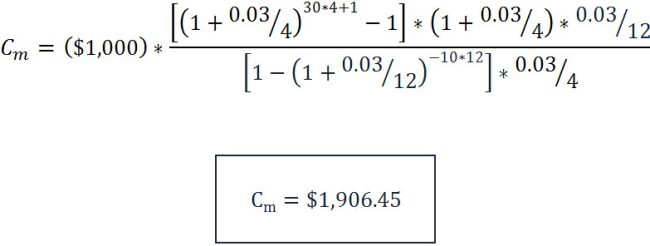So, with \$500,000 and a rate of 3.25%, you would receive a monthly income of \$4,886 for the next ten years.

## How to calculate payments for single-premium, deferred fixed annuities

In the case of deferred annuities, there are several different scenarios. One is when you pay a single premium to the insurance company, and your money grows at a certain rate before the annuitization phase. Another case is when you make periodic payments to the insurance company like you would add contributions to a 401(k), and a third case would be a combination of both.

In any of the three cases, payments can be calculated with the same equation as above by replacing A0 with the future value of all of your deposits by the time the annuity reaches maturity (when you retire).

In the case of single premium, deferred annuities, the future value of your premium is calculated with the equation of compounding interest: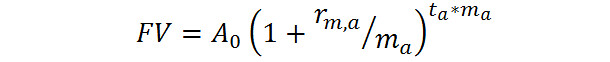Here, rm,a represents the rate during the accumulation phase and ta is the number of years your investment grows before the payout or annuitization phase. Remember, this future value will actually be the "present" value of your investment once payments commence (which we'll call A'0). So, the income you can expect to receive will be: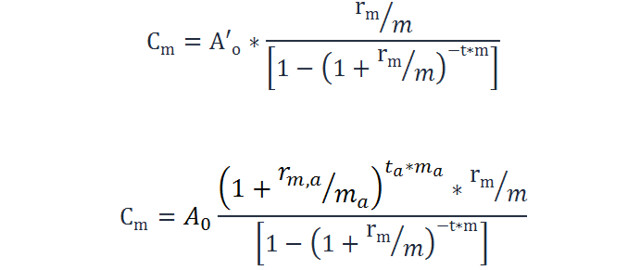Where A0 is your principal (the lump sum you pay the insurance company when you sign the contract); rm,a and rm are the annual rates during the accumulation and annuitization phases, respectively; ta is the number of years your money grows before the annualization phase (the length of the accumulation phase) and t is the number of years you'll receive your guaranteed income (the same as t in the previous equation).

### Example #2: Income from a \$100,000 10-year deferred annuity purchased at age 30 with payouts beginning at age 60, and a constant rate of 3%

If you're 30 and want to know how much income you can get when you turn 60 by purchasing a \$100,000 deferred annuity today that grows at a constant 3% rate both before and after the payout phase, you use the equation above.

In this case, this is the information we have:

• ma = m = 12.
• The rates are rm,a = rm = r12 = 3% = 0.03
• A0 = \$100.000
• You're 30 now, and you expect to receive a fixed income for ten years after turning 60, so ta = 60 – 30 = 30 years, and t = 10 years.
• Cm = C12 = ?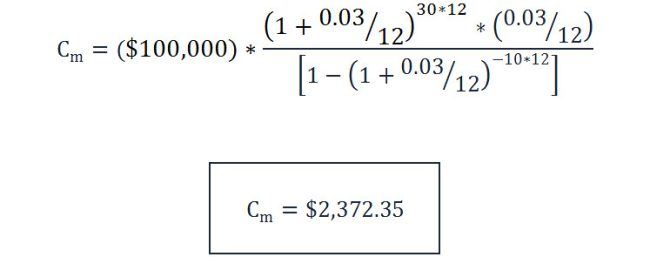Therefore, if you're 30 and invest \$100,000 today, and start receiving payments when you turn 60, you can expect to receive \$2,372 per month until you turn 70.

## How to calculate payments for deferred fixed annuities with periodic contributions

In the case of a deferred annuity where you make consecutive monthly, bi-monthly, quarterly or annual payments during the accumulation phase, the payout is calculated based on the future value of your savings once the annuitization phase begins (in other words, the amount of principal you will have saved by the time payouts begins, plus interests).

Assuming you make the first payment the day you sign the contract with the insurance company, by the day the accumulation period ends, you'll have the following amount, calculated as the future value of an annuity due with p+1 compounding periods: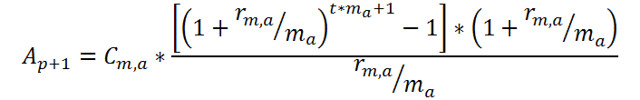Once again, this will be equal to A'0 when payouts begin. So, the payments will be equal to: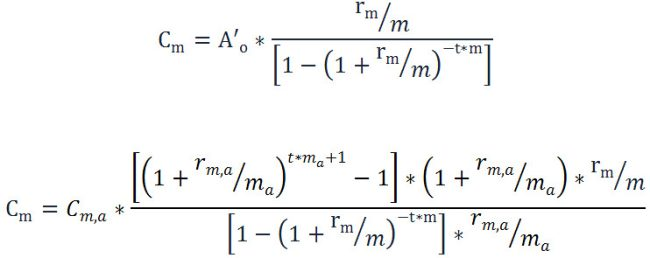Using these equations can help even beginners budget effectively over the long run since they give clear guidance on how much to set aside every month for a comfortable retirement.

### Example #3: Income from a 10-year deferred annuity purchased at age 30 by making quarterly payments of \$1,000, with payouts beginning at age 60, and a constant rate of 3%

In this example, you enter an annuity contract where you don't pay a single lump sum at the beginning but rather start making deposits of \$1,000 every quarter starting immediately when you're 30. By the time you retire and turn 60, you start receiving payments from the insurance company.

In this case, this is the information we have:

• ma = 4 (because you make quarterly contributions during the accumulation phase)
• m = 12 (because you still expect to get a monthly income, not a quarterly income)
• The rates are rm,a = rm = 3% = 0.03
• Cm,a = C4,a = \$1.000 (the payments or premiums you pay quarterly)
• You're 30 now, and you expect to receive a fixed income for ten years after turning 60, so ta = 60 – 30 = 30 years, and t = 10 years.
• Cm = C12 = ?

So, by saving \$1,000 every quarter from age 30 to 60, you'll guarantee an income of \$1,906 per month for ten years.

## The bottom line

Calculating annuity income isn't hard to do, but there are many things to consider. This is just a sample of calculations in the simplest of cases, but they can help you grasp what calculating annuity income is all about. When you buy into a contract, the numbers may not agree with your calculations entirely, mostly because of fees we didn't consider here.

However, you should still be able to use the formulas shown in this post to get a very close estimate of how much you can expect to receive in the future from what you save now.

The post How is An Annuity Income Payout Calculated? appeared first on Due.